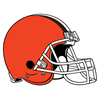DraftKings 2019 AFC Championship Odds
+120
1.2 to 1Ravens
20.6% implied probability

+160
1.6 to 1Patriots
17.4% implied probability

+550
5.5 to 1Chiefs
7.0% implied probability

+1200
12 to 1Texans
3.5% implied probability

+2000
20 to 1Bills
2.2% implied probability

+3300
33 to 1Titans
1.3% implied probability

+4000
40 to 1Steelers
1.1% implied probability

+8000
80 to 1Colts
0.6% implied probability

+10000
100 to 1Raiders
0.4% implied probability

+15000
150 to 1Browns
0.3% implied probability

+25000
250 to 1Chargers
0.2% implied probability

+35000
350 to 1Broncos
0.1% implied probability

+50000
500 to 1Jaguars
0.1% implied probability

+50000
500 to 1Jets
0.1% implied probability

+250000
2500 to 1Dolphins
0.0% implied probability